Ex 8.2

Chapter 8 Class 11 Sequences and Series
Serial order wise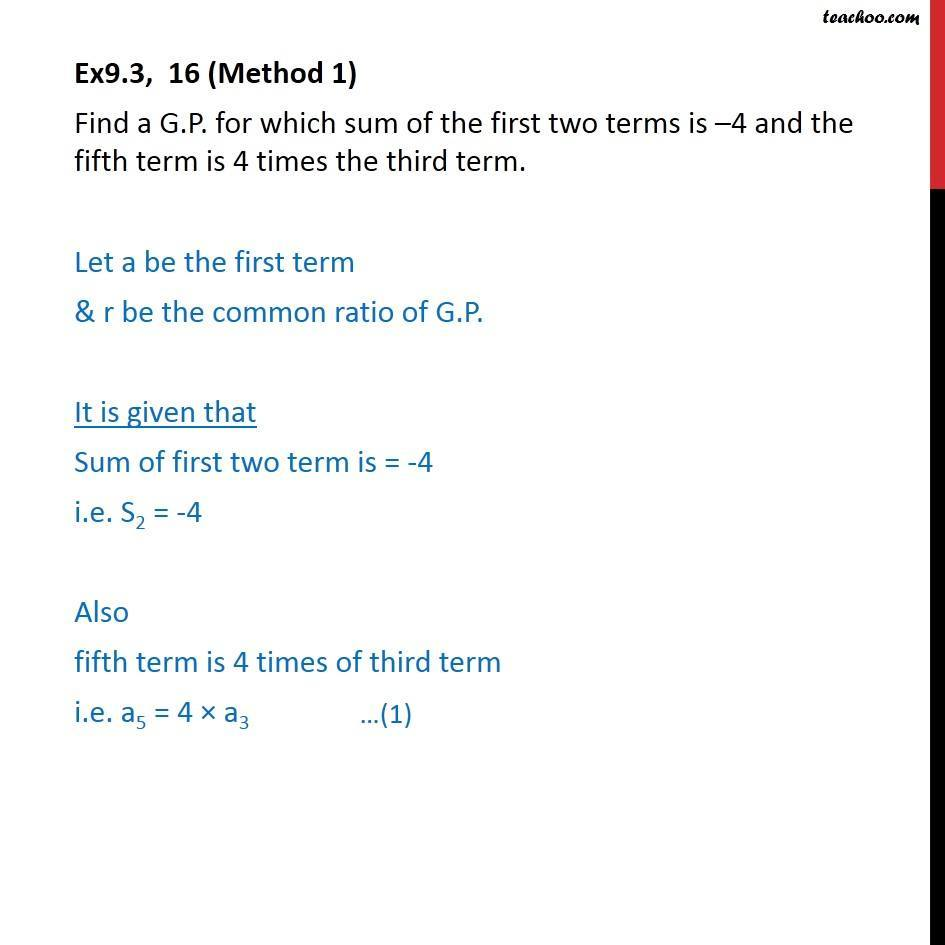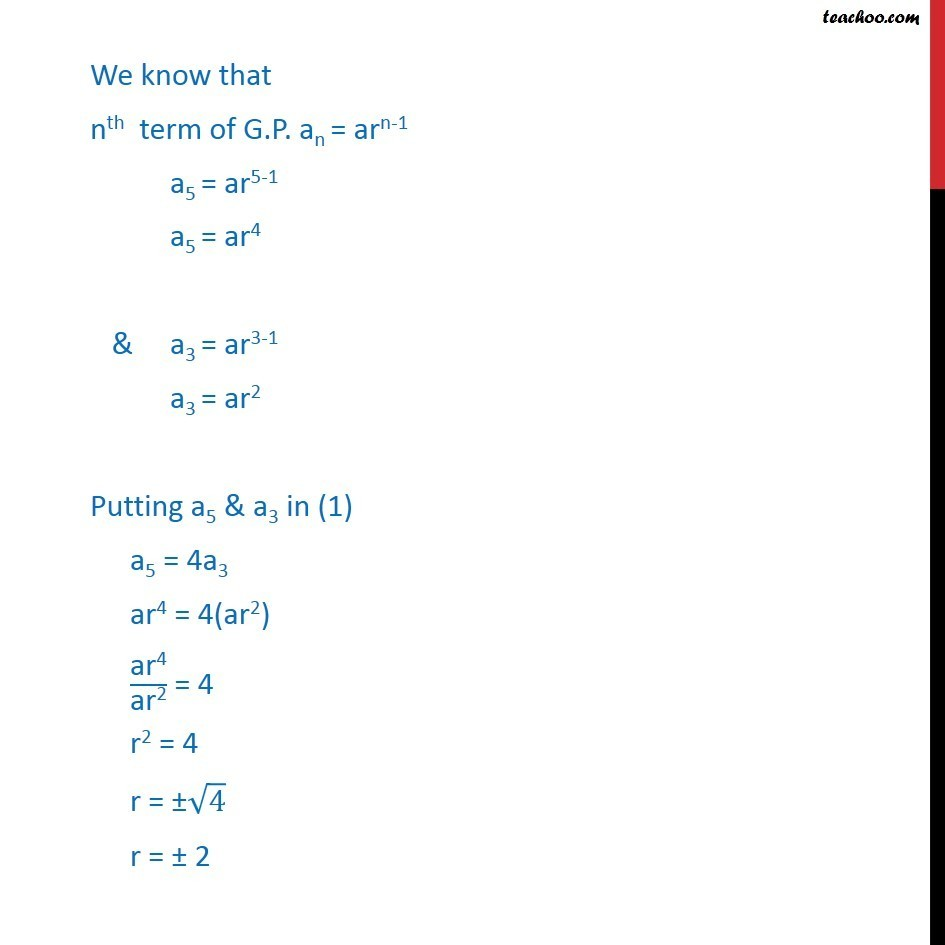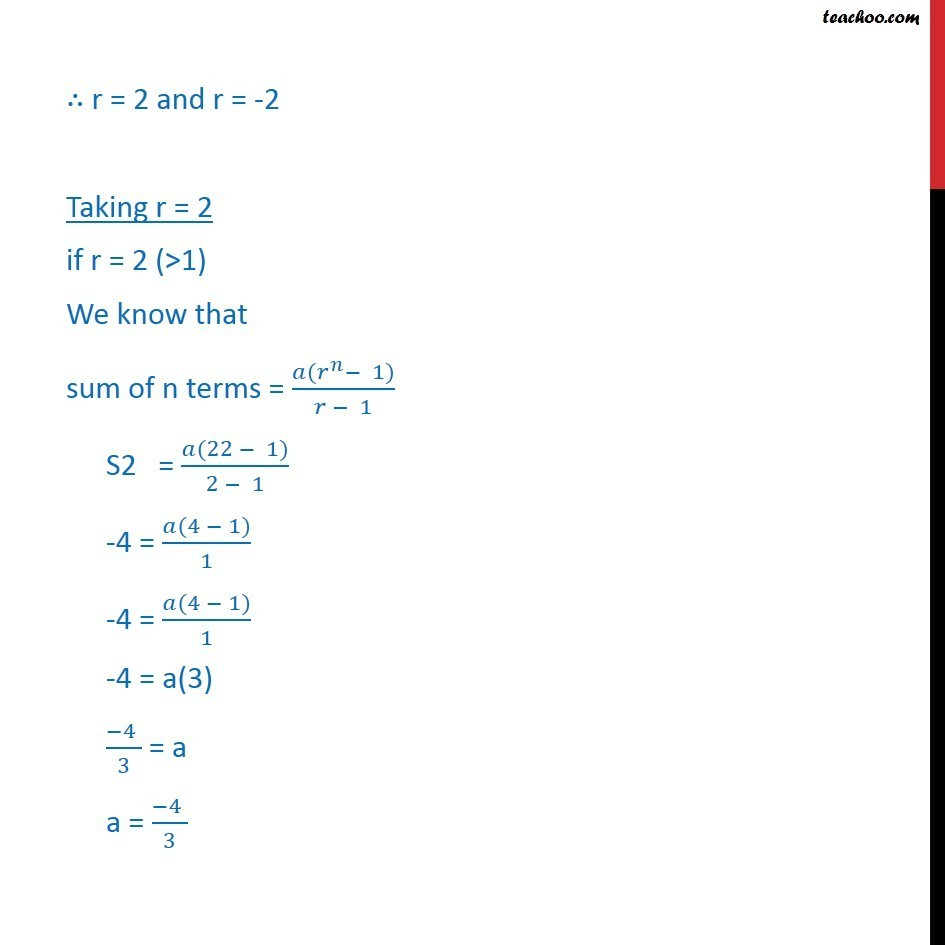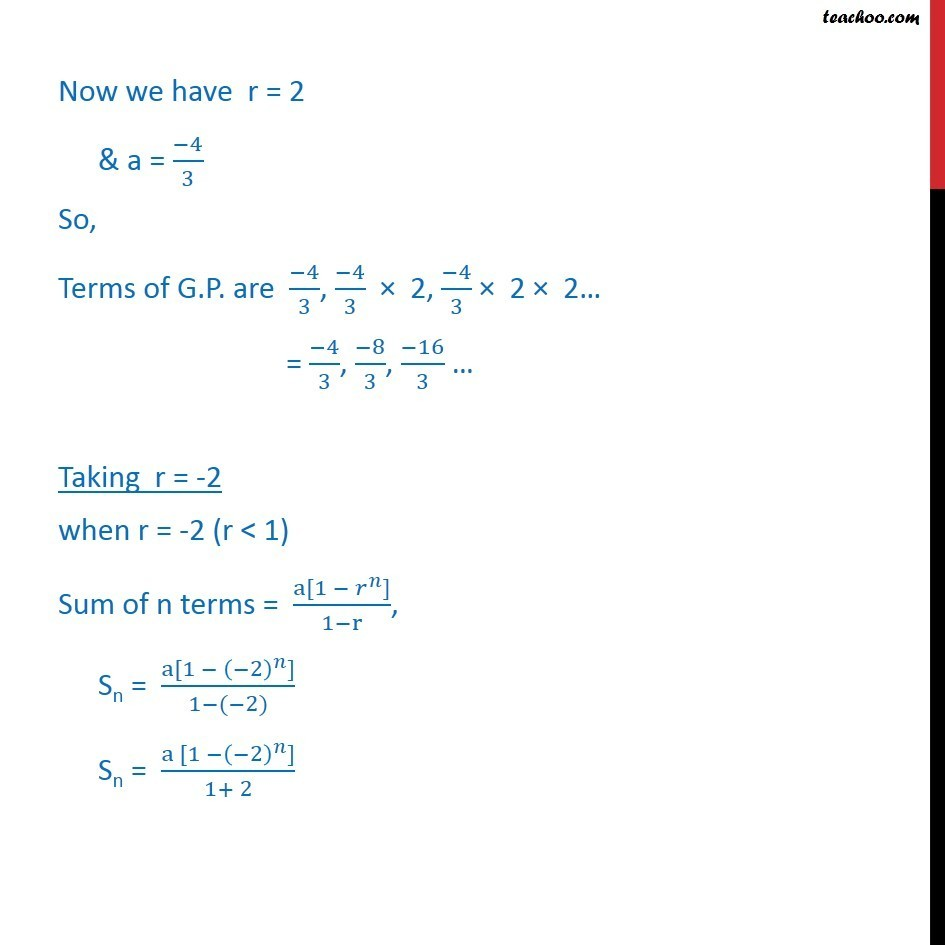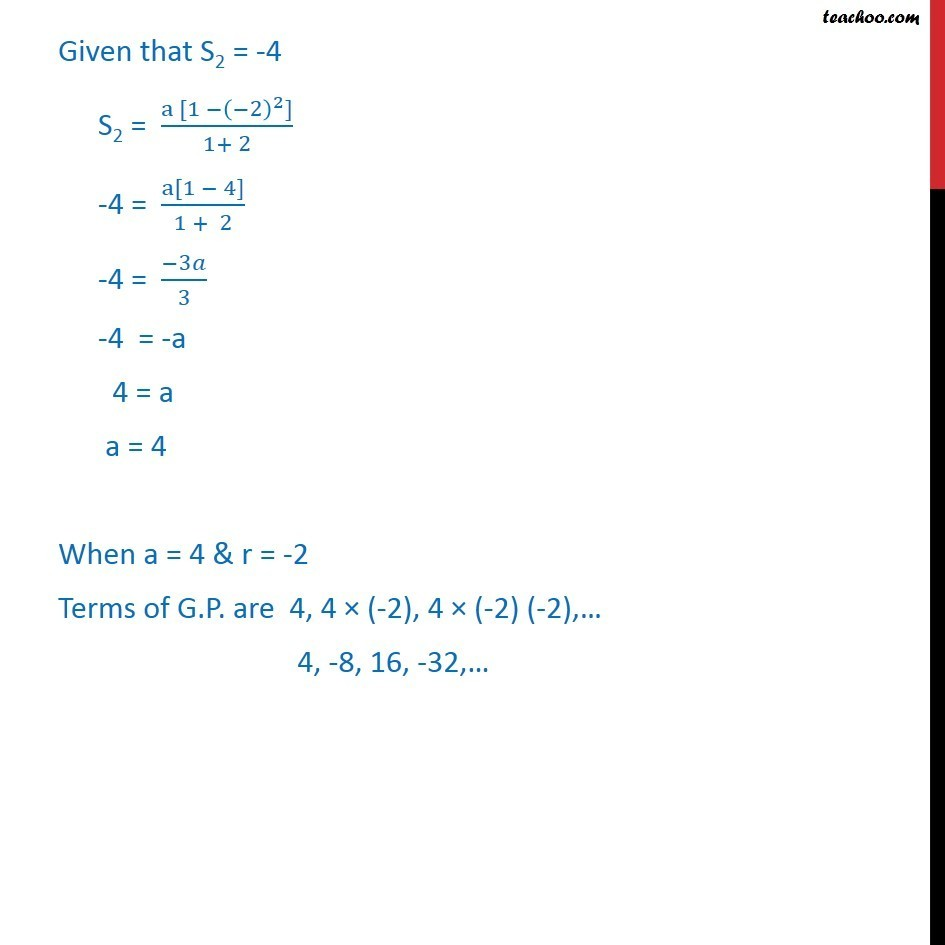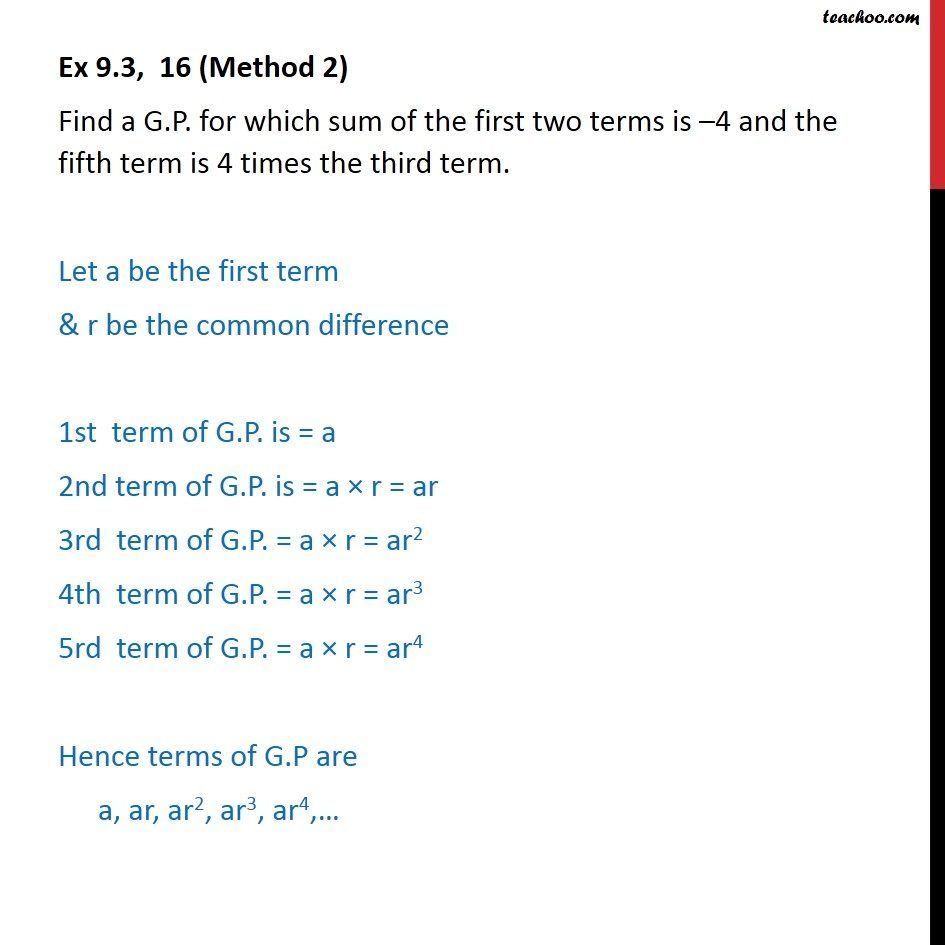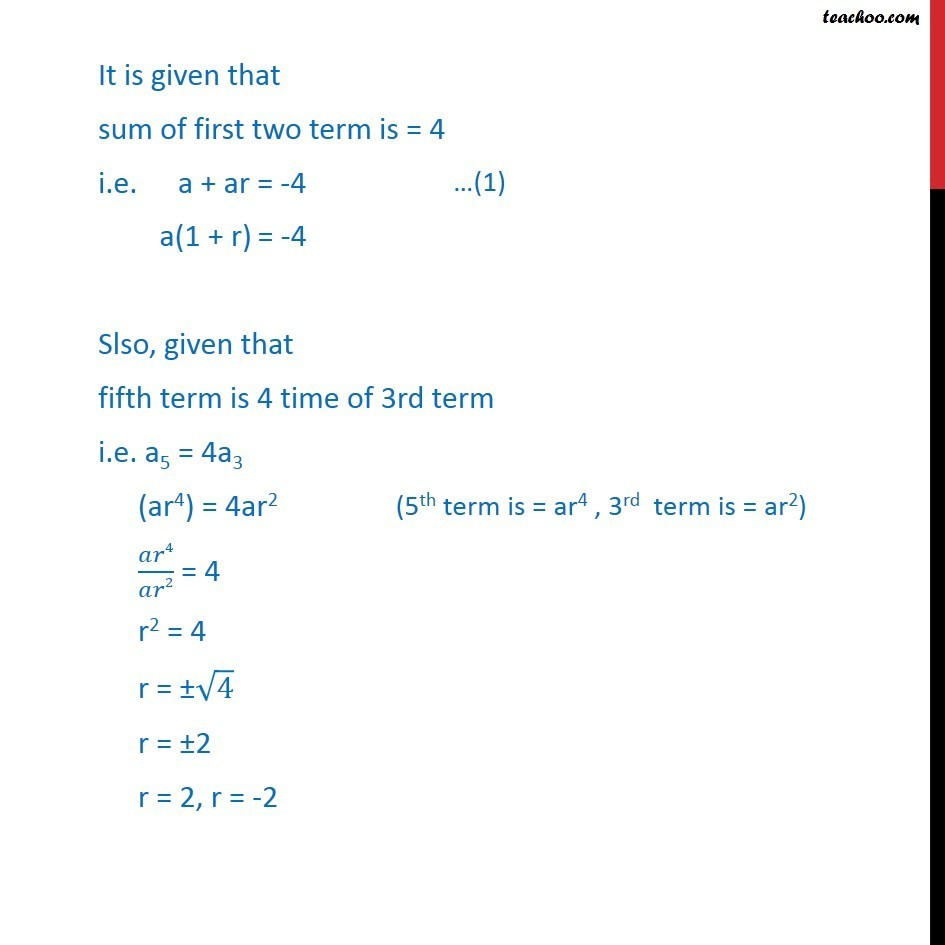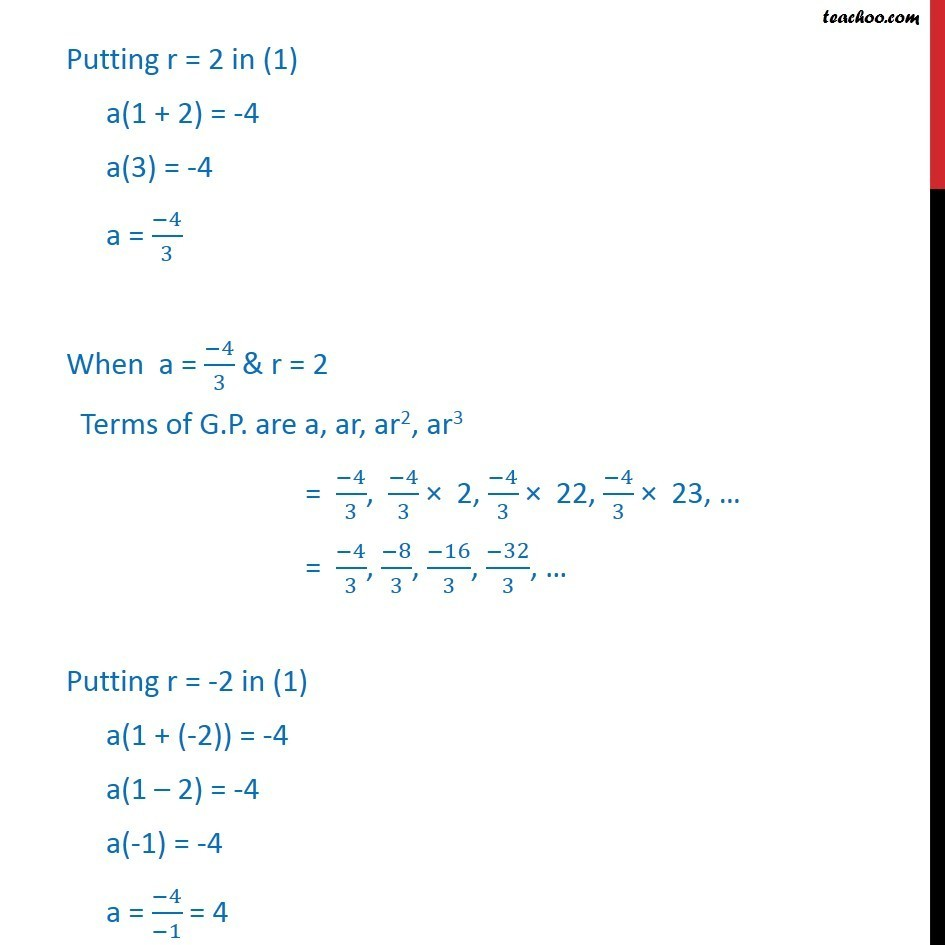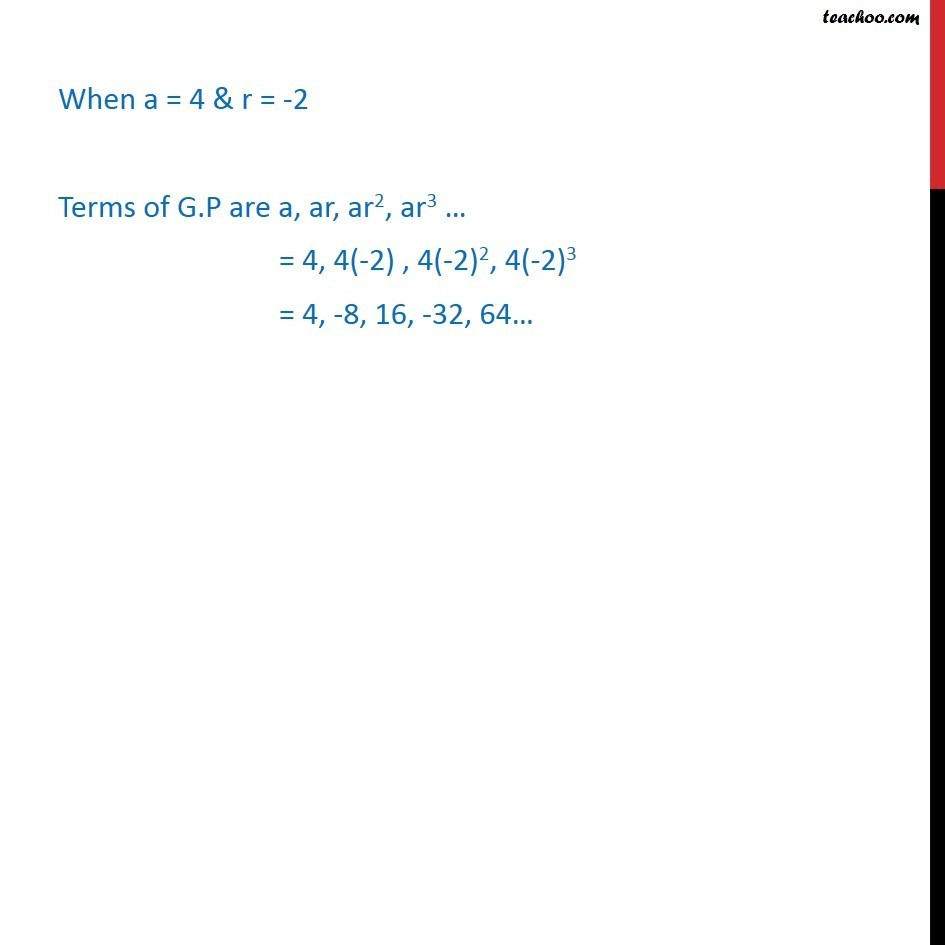Learn in your speed, with individual attention - Teachoo Maths 1-on-1 Class

### Transcript

Ex9.3, 16 (Method 1) Find a G.P. for which sum of the first two terms is –4 and the fifth term is 4 times the third term. Let a be the first term & r be the common ratio of G.P. It is given that Sum of first two term is = -4 i.e. S2 = -4 Also fifth term is 4 times of third term i.e. a5 = 4 × a3 We know that nth term of G.P. an = arn-1 a5 = ar5-1 a5 = ar4 & a3 = ar3-1 a3 = ar2 Putting a5 & a3 in (1) a5 = 4a3 ar4 = 4(ar2) "ar4" /"ar2" = 4 r2 = 4 r = ±√4 r = ± 2 ∴ r = 2 and r = -2 Taking r = 2 if r = 2 (>1) We know that sum of n terms = (𝑎(𝑟^𝑛− 1))/(𝑟 − 1) S2 = (𝑎(22 − 1))/(2 − 1) -4 = (𝑎(4 − 1))/1 -4 = (𝑎(4 − 1))/1 -4 = a(3) (−4 )/3 = a a = (−4 )/3 Now we have r = 2 & a = (−4)/3 So, Terms of G.P. are (−4)/3, (−4)/3 × 2, (−4)/3 × 2 × 2… = (−4)/3, (−8)/3, (−16)/3 … Taking r = -2 when r = -2 (r < 1) Sum of n terms = (a[1 − 𝑟^𝑛])/(1−r), Sn = (a[1 − (−2)^𝑛])/(1−(−2)) Sn = (a [1 −(−2)^𝑛])/(1+ 2) Given that S2 = -4 S2 = (a [1 −(−2)^2])/(1+ 2) -4 = (a[1 − 4])/(1 + 2) -4 = (−3𝑎)/3 -4 = -a 4 = a a = 4 When a = 4 & r = -2 Terms of G.P. are 4, 4 × (-2), 4 × (-2) (-2),… 4, -8, 16, -32,… Ex 8.2, 16 (Method 2) Find a G.P. for which sum of the first two terms is –4 and the fifth term is 4 times the third term. Let a be the first term & r be the common difference 1st term of G.P. is = a 2nd term of G.P. is = a × r = ar 3rd term of G.P. = a × r = ar2 4th term of G.P. = a × r = ar3 5rd term of G.P. = a × r = ar4 Hence terms of G.P are a, ar, ar2, ar3, ar4,… It is given that sum of first two term is = 4 i.e. a + ar = -4 a(1 + r) = -4 Slso, given that fifth term is 4 time of 3rd term i.e. a5 = 4a3 (ar4) = 4ar2 𝑎𝑟4/𝑎𝑟2 = 4 r2 = 4 r = ±√4 r = ±2 r = 2, r = -2 Putting r = 2 in (1) a(1 + 2) = -4 a(3) = -4 a = (−4)/3 When a = (−4)/3 & r = 2 Terms of G.P. are a, ar, ar2, ar3 = (−4)/3, (−4)/3 × 2, (−4)/3 × 22, (−4)/3 × 23, … = (−4)/3, (−8)/3, (−16)/3, (−32)/3, … Putting r = -2 in (1) a(1 + (-2)) = -4 a(1 – 2) = -4 a(-1) = -4 a = (−4)/(−1) = 4 When a = 4 & r = -2 Terms of G.P are a, ar, ar2, ar3 … = 4, 4(-2) , 4(-2)2, 4(-2)3 = 4, -8, 16, -32, 64…